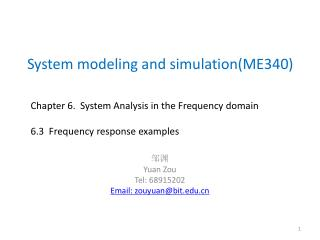# System modeling and simulation(ME340) - PowerPoint PPT PresentationDownload PresentationSystem modeling and simulation(ME340)

System modeling and simulation(ME340)Download Presentation## System modeling and simulation(ME340)

- - - - - - - - - - - - - - - - - - - - - - - - - - - E N D - - - - - - - - - - - - - - - - - - - - - - - - - - -
##### Presentation Transcript

1. System modeling and simulation(ME340) Chapter6.System Analysis in the Frequency domain 6.3 Frequency response examples 邹渊 Yuan Zou Tel: 68915202 Email: zouyuan@bit.edu.cn

2. The neutrally stable case • ??? Consider Mass-spring-damping system when damping c=0 • mx’’+kx=Fsin(wt)

3. Beating • Just consider the forced response:

4. Explain this force response.

5. And this

6. What happed if r is 1?

7. Resonance and transient response • 4x’’+3x’+100x=295*sin(0.7t*t).

8. Two-mass suspension model

9. Bode plot

10. System modeling and simulation(ME340) Chapter6.System Analysis in the Frequency domain 6.4 Filter property of dynamic system 邹渊 Yuan Zou Tel: 68915202 Email: zouyuan@bit.edu.cn

11. Filtering properties of dynamic system • Dynamic systems response in a different way to the input with the different frequency. • Low-pass: Vehicle suspension? • High-pass: RC circuit?

12. Bandwidth • The range of frequencies over which the power transmitted or dissipated by the system is no less than one-half of the peak power. • [w1,w2]

13. Bandwidth of first-order system • Band width: [0, 1/];

14. Band width of second-order system

15. Different dynamic behavior

16. Alternative definition of bandwidth • Often the low-frequency input might account for more power than those of high-frequency input. • Sometimes bandwidth is defined as [0, ]. • But It is not true for high-pass filter system.

17. Discussion for Human-ears property